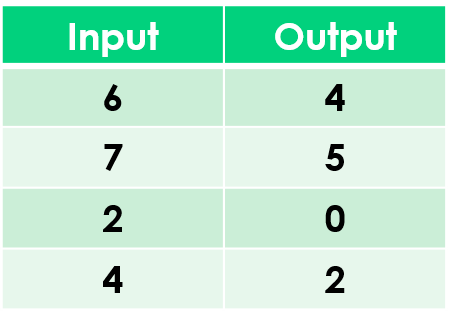1
visibility

Find the rule from the table below:• A

Subtract 2

• B

Subtract 4

The correct answer is "Subtract 2".

Please Note:
Subtraction is the operation that decreases a number by taking away from it.

Solution:
To find the correct rule, we consider the given table.

We observe that the given rule makes each number decrease. This implies subtraction.

We try subtracting 2 from each input number:

6 - 2 = 4
7 - 2 = 5
2 - 2 = 0
4 - 2 = 2

On subtracting 2 from the input numbers, we get the corresponding output numbers.

So, the rule is “subtract 2”.